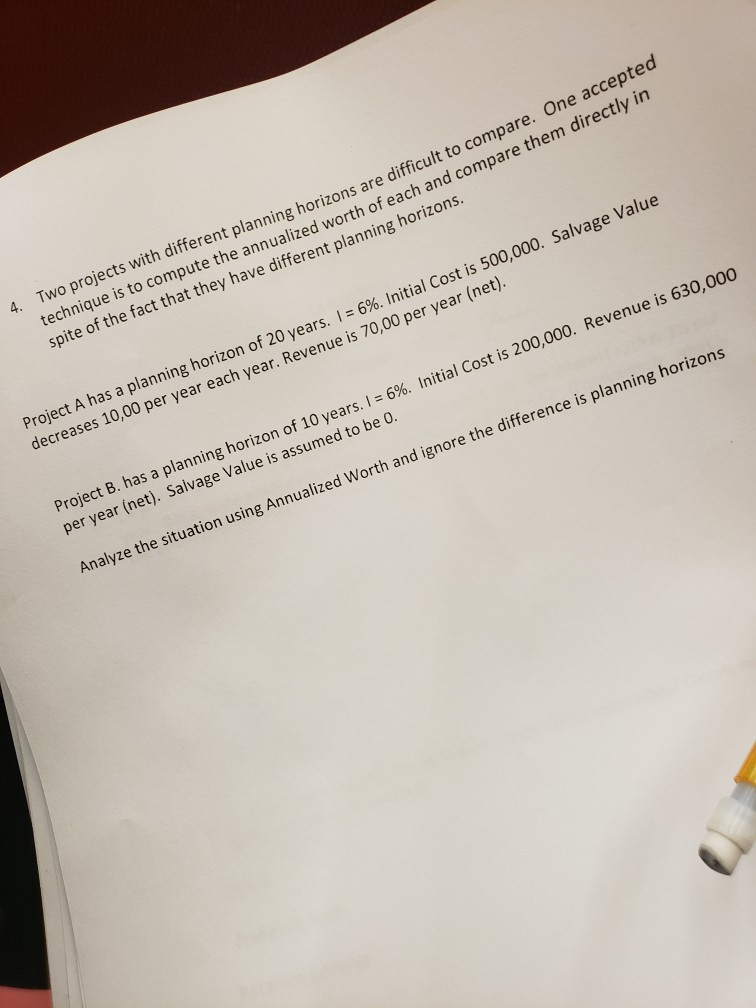Homework Help Question & Answers

# E accepted Two projects with different planning horizons are difficult to compare. On technique i...e accepted Two projects with different planning horizons are difficult to compare. On technique is to compute the annualized worth of each and compare them directly in spite of the fact that they have different planning horizons. 4. Project A has a planning horizon of 20 years. 1-6%. Initial Cost is 500,000. Salvage Value decreases 10,00 per year each year. Revenue is 70,00 per year (net), Project B. has a planning horizon of 10 years. I-696. Initial Cost is 200,000. Revenue is 630,000 per year (net). Salvage Value is assumed to be o. Analyze the situation using Annualized Worth and ignore the difference is planning horizons

#### Homework Answers

ReportAnswer #1

Project A:

Initial cost = 500,000

Salvage value at the end of the year 20: 500,000 – (20*1000)

= 500,000 – 20,000

= 480,000

Net Revenue: 70,00

Interest: 6%

Time Period: 20 years

Computation of NPV and annualized worth

1. Initial outflow: 500,000
2. Net cash flow: 7000 per year
3. Terminal Cash inflow (salvage value at the end of the project) : 480,000
 Year cash flow Present value factor Present value of cash flow 0 -500000 1 -500000 1 to 20 7000 11.47 80290 20 480000 0.312 149760 Net Present Value 269950 Present value annuity factor 11.47 Annualised worth 23535.31

Therefore,, the annualised worth of project A is 23535.31.

Project B:

Initial cost = 200,000

Salvage value at the end of the year 10: 0

Net Revenue: 630000

Interest: 6%

Time Period: 10 years

Computation of NPV and annualized worth

1. Initial outflow: 200,000
2. Annual cash flow during the term of the project: 630,000
3. Terminal Cash inflow (salvage value at the end of the project) :
 Year cash flow Present value factor Present value of cash flow 0 -200000 1 -200000 1 to 10 630000 7.36 46,36,800 10 0 0 0 Net Present Value 4436800 Present value annuity factor 7.36 Annualised worth 602826.1

Therefore the annualised worth of project b is 602826.1.

By comparing the annualised worth of project A and B, we find that the Project B is better than Project A. Hence, project B should be choosen.

Add Homework Help Answer
##### Add Answer of: E accepted Two projects with different planning horizons are difficult to compare. On technique i...
Your Answer: Your Name: What's your source?
Not the answer you're looking for? Ask your own homework help question. Our experts will answer your question WITHIN MINUTES for Free.
More Homework Help Questions Additional questions in this topic.

• #### Use the discriminant to determine whether the following equations have solutions that are: two different rational solutions; two different irrational solutions; exactly one rational solution; or two different imaginary solutions

Need Online Homework Help?

Get FREE EXPERT Answers
WITHIN MINUTES
Related Questions